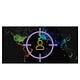# Understanding RSA Cryptosystem

RSA is named after its inventors, Ron Rivest, Adi Shamir, and Len Adleman.
Each person needs to generate a pair of keys to communicate using RSA encryption.

We’ll take a look at how to about it and what goes into the process.

# How is each RSA Key pair generated ?

Generate the RSA modulus (n)

• Select two large primes, p and q.
• Calculate n=p*q.
For strong unbreakable encryption, let n be a large number, typically a minimum of 512 bits

Find Derived Number (e)

• Number e must be greater than 1 and less than (p − 1)(q − 1).
• There must be no common factor for e and (p − 1)(q − 1) except for 1.
In other words two numbers e and (p — 1)(q — 1) are coprime.

Formation of the public key

• The pair of numbers (n, e) form the RSA public key and is made public.
• Interestingly, though n is part of the public key, difficulty in factorizing a large prime number ensures that attacker cannot find in finite time the two primes (p & q) used to obtain n.
This is the core strength of RSA.

Generate the private key

• Private Key d is calculated from p, q, and e. For given n and e, there is unique number d.
• Number d is the inverse of e modulo (p — 1)(q — 1). This means that d is the number less than (p — 1)(q — 1) such that when multiplied by e, it is equal to 1 modulo (p — 1)(q — 1).
• This relationship is written mathematically as follows −
`ed = 1 mod (p − 1)(q − 1)`

The Extended Euclidean Algorithm takes p, q, and e as input and gives d as output.

For ease of understanding, the primes p & q taken here are small values. Practically, these values are very high.

• Let two primes be p = 13 and q = 43. Thus, modulus n = pq = 13 x 43 = 559.
• Select e = 5, which is a valid choice since there is no number that is common factor of 5 and (p − 1)(q − 1) = 6 × 12 = 72, except for 1.
• The pair of numbers (n, e) = (91, 5) forms the public key and can be made available to anyone whom we wish to be able to send us encrypted messages.
• Input p = 7, q = 13, and e = 5 to the Extended Euclidean Algorithm.
The output will be d = 29.
• Check that the d calculated is correct by computing
`de = 29 × 5 = 145 = 1 mod 72`

Hence, public key is (91, 5) and private keys is (91, 29).

• Suppose the sender wish to send some text message to someone whose public key is (n, e).
• The sender then represents the plaintext as a series of numbers less than n.
• To encrypt the first plaintext P, which is a number modulo n. The encryption process is simple mathematical step as -
`C = P^e mod n`
• In other words, the ciphertext C is equal to the plaintext P multiplied by itself e times and then reduced modulo n. This means that C is also a number less than n.

Returning to our Key Generation example with plaintext P = 10, we get ciphertext C

• Suppose that the receiver of public-key pair (n, e) has received a ciphertext C.
• Receiver raises C to the power of his private key d. The result modulo n will be the plaintext P.
`Plaintext = Cd mod n`

Returning to our numerical example, the ciphertext C = 82 would get decrypted to number 10 using private key 29

`Plaintext = 8229 mod 91 = 10`

The security of RSA depends on the strengths of two separate functions. The RSA cryptosystem is most popular public-key cryptosystem strength of which is based on the practical difficulty of factoring the very large numbers.

• Encryption Function :
It is considered a one-way function of converting plaintext into ciphertext and can only be reversed with knowledge of private key d.
• Key Generation :
The difficulty of determining a private key from an RSA public key is equivalent to factoring the modulus n.

An attacker thus cannot use knowledge of an RSA public key to determine an RSA private key unless he can factor n. It is also a one way function, going from p & q values to modulus n is easy but reverse is not possible.

If either of these two functions are proved to not be one-way functions, then RSA will be broken.

The strength of RSA encryption dramatically goes down against attacks if the number p and q are not large primes and/ or chosen public key e is a small number.

This article bears significant resemblance to an article on tutorialspoint on the RSA Algorithm.
Most articles I write are an amalgate of the information I find in public domain. The copyright of content does belong to their original owners.

Reference:

Cheers!

Written by

## Crypto-0-nite

Written by

#### Protagonist. Freedom. Bitcoin.